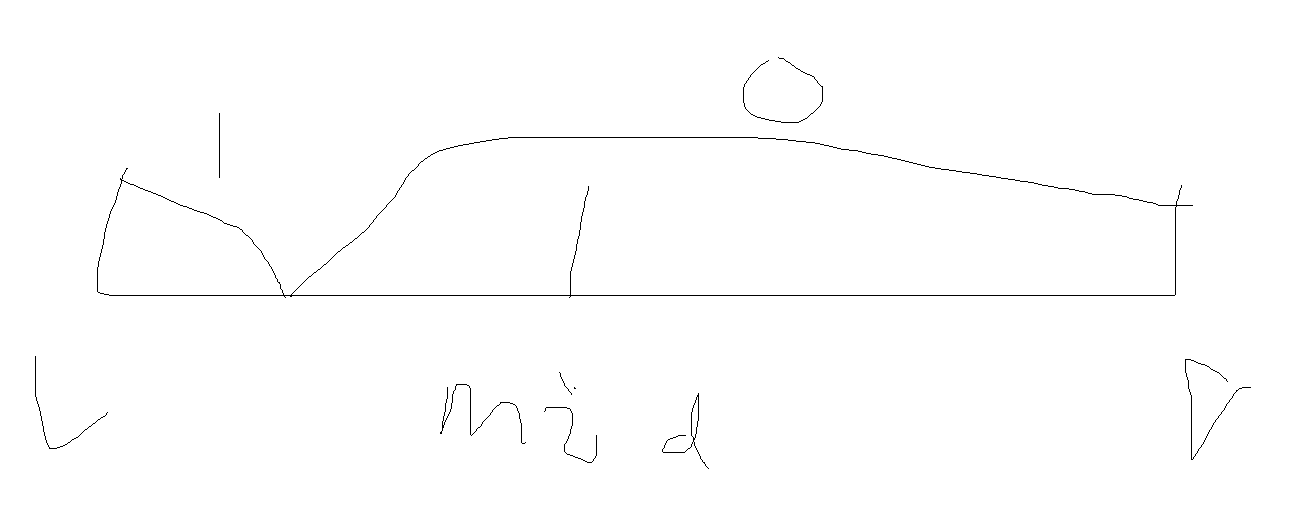## 社团活动

### Description

bbq的工作是管理学校的社团活动，具体来说是为每个社团活动分配教室。要把有限的教室合理安排给这些社团，是不容易的。

bbq总是按社团活动申请的先后顺序分配教室，如果某一天剩余的教室数量不够满足某社团的要求，则停止教室的分配。bbq需要告知该社团，他们的该次社团活动无法进行。

### Input

`第二行包含n个正整数，其中第i个数为r`
i
`，表示第i天空教室的数量。`

`1≤n, m≤10`
6
`，0≤ri≤10`
9
`，0≤k≤109  ,1≤t1≤t2≤n。`

```4 3
2 5 4 3
2 1 3
3 2 4
4 2 4
```

### Sample Output

```2
``````#include<iostream>
#include<cstring>
using namespace std;
const int N=1e6+50;
long long n,m;
long long a[N],room[N],t1[N],t2[N],sum[N];
int check(int mid){
memset(sum,0,sizeof(sum));
for(int i=1;i<=mid;i++){
sum[t1[i]]-=room[i];
sum[t2[i]+1]+=room[i];
}
int cnt=0;
for(int i=1;i<=n;i++){
cnt+=sum[i];
if(a[i]+cnt<0)
return 0;
}
return 1;
}
int main(){
cin>>n>>m;
for(int i=1;i<=n;i++){
cin>>a[i];
}
for(int i=1;i<=m;i++){
cin>>room[i]>>t1[i]>>t2[i];
}
int l=1,r=m+1;
while(l<r){
int mid=(l+r)>>1;
if(check(mid))
l=mid+1;
else
r=mid;
}
if(l==m+1)
cout<<0<<endl;
else
cout<<l<<endl;
return 0;
} ```
View Code

使用二分答案需要找到答案区间的最小值l和最大值r，然后用（l+r)>>1来表示mid，(l+r)>>1的意思是，(l+r)除以2的整数部分，然后呢还需要一个check函数，将mid传进这个函数里，这个函数来判断mid是否符合条件，二分答案中，check函数的书写是比较重要的，check函数也会因题目而异，二分答案的其他部分就可以模板化了如果mid所指的活动没有被满足（如上图），我们就r=mid，这时候就要改变右边界的界限，注意，因为mid是不被满足的，而我们要找的就是不被满足的，所以我们不能丢掉mid，如果用r=mid-1，那就错了。